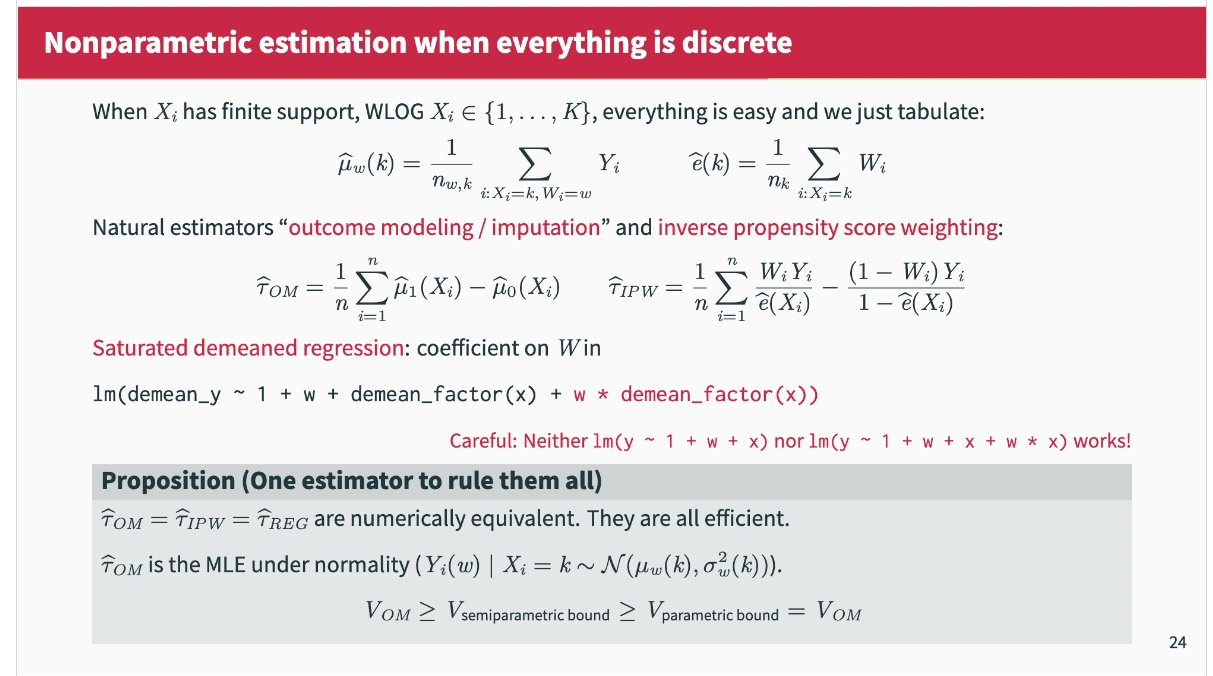# Tuning-Free Covariate Adjustment with Discrete Covariates

causal
experiments
Author

Apoorva Lal

Published

August 1, 2022

$$\DeclareMathOperator*{\argmin}{argmin}$$ $$\newcommand{\norm}{\left\Vert{#1} \right\Vert}$$ $$\newcommand{\var}{\mathrm{Var}}$$ $$\newcommand{\tra}{^{\top}}$$ $$\newcommand{\sumin}{\sum_{i=1}^n}$$ $$\newcommand{\sumiN}{\sum_{i=1}^n}$$ $$\newcommand{\epsi}{\varepsilon}$$ $$\newcommand{\phii}{\varphi}$$ $$\newcommand\Bigpar{\left( #1 \right )}$$ $$\newcommand\Bigbr{\left[ #1 \right ]}$$ $$\newcommand\Bigcr{\left\{ #1 \right \}}$$ $$\newcommand\SetB{\left\{ #1 \right\}}$$ $$\newcommand\Sett{\mathcal{#1}}$$ $$\newcommand{\Data}{\mathcal{D}}$$ $$\newcommand{\E}{\mathbb{E}}$$ $$\newcommand{\Ubr}{\underbrace{#1}_{\text{#2}}}$$ $$\newcommand{\Obr}{ \overbrace{#1}^{\text{#2}}}$$ $$\newcommand{\sumiN}{\sum_{i=1}^N}$$ $$\newcommand{\dydx}{\frac{\partial #1}{\partial #2}}$$ $$\newcommand\Indic{\mathbb{1}_{#1}}$$ $$\newcommand{\Realm}{\mathbb{R}^{#1}}$$ $$\newcommand{\Exp}{\mathbb{E}\left[#1\right]}$$ $$\newcommand{\Expt}{\mathbb{E}_{#1}\left[#2\right]}$$ $$\newcommand{\Var}{\mathbb{V}\left[#1\right]}$$ $$\newcommand{\Covar}{\text{Cov}\left[#1\right]}$$ $$\newcommand{\Prob}{\mathbf{Pr}\left(#1\right)}$$ $$\newcommand{\Supp}{\text{Supp}\left[#1\right]}$$ $$\newcommand{\doyx}{\Prob{Y \, |\,\mathsf{do} (X = x)}}$$ $$\newcommand{\doo}{\Prob{Y |\,\mathsf{do} (#1) }}$$ $$\newcommand{\R}{\mathbb{R}}$$ $$\newcommand{\Z}{\mathbb{Z}}$$ $$\newcommand{\wh}{\widehat{#1}}$$ $$\newcommand{\wt}{\widetilde{#1}}$$ $$\newcommand{\wb}{\overline{#1}}$$ $$\newcommand\Ol{\overline{#1}}$$ $$\newcommand\Ul{\underline{#1}}$$ $$\newcommand\Str{#1^{*}}$$ $$\newcommand{\F}{\mathsf{F}}$$ $$\newcommand{\ff}{\mathsf{f}}$$ $$\newcommand{\Cdf}{\mathbb{F}\left(#1\right)}$$ $$\newcommand{\Cdff}{\mathbb{F}_{#1}\left(#2\right)}$$ $$\newcommand{\Pdf}{\mathsf{f}\left(#1\right)}$$ $$\newcommand{\Pdff}{\mathsf{f}_{#1}\left(#2\right)}$$ $$\newcommand{\dd}{\mathsf{d}}$$ $$\newcommand\Normal{\mathcal{N} \left( #1 \right )}$$ $$\newcommand\Unif{\mathsf{U} \left[ #1 \right ]}$$ $$\newcommand\Bern{\mathsf{Bernoulli} \left( #1 \right )}$$ $$\newcommand\Binom{\mathsf{Bin} \left( #1 \right )}$$ $$\newcommand\Pois{\mathsf{Poi} \left( #1 \right )}$$ $$\newcommand\BetaD{\mathsf{Beta} \left( #1 \right )}$$ $$\newcommand\Diri{\mathsf{Dir} \left( #1 \right )}$$ $$\newcommand\Gdist{\mathsf{Gamma} \left( #1 \right )}$$ $$\def\mbf#1{\mathbf{#1}}$$ $$\def\mrm#1{\mathrm{#1}}$$ $$\def\mbi#1{\boldsymbol{#1}}$$ $$\def\ve#1{\mbi{#1}}$$ $$\def\vee#1{\mathbf{#1}}$$ $$\newcommand{\Mat}{\mathbf{#1}}$$ $$\newcommand{\eucN}{\norm{#1}}$$ $$\newcommand{\lzero}{\norm{#1}_0}$$ $$\newcommand{\lone}{\norm{#1}_1}$$ $$\newcommand{\ltwo}{\norm{#1}_2}$$ $$\newcommand{\pnorm}{\norm{#1}_p}$$

## Covariate Adjustment on Compressed Data

Let $$\{Y_i, W_i, X_i\}_{i=1}^N$$ be IID draws, where $$W_i \in \{0, 1, \dots, K\}$$ is a treatment assignment from a finite set, $$Y_i \in \R$$ is a scalar outcome, and $$X_i \in \mathcal{X}$$ is a set of discrete covariates. wlog, I focus on the two-treatment case. Throughout, we make the standard assumptions of

1. Consistency: $$Y_i = W_i Y^1_i + (1-W_i) Y^0_i$$
2. Unconfoundedness: $$Y^1 , Y^0 \bot W | X$$
3. Overlap: $$0 < P(W = 1 | X) < 1$$

The AIPW estimator of Hahn (1998) involves fitting three nonparametric regressions:

$\wh{\E}[W_i Y_i | X_i = x_i] = \frac{\sum_i W_i Y_i 1(X_i = x_i)}{ \sum_i 1(X_i = x_i)}$

$\wh{\E}[(1-W_i) Y_i | X_i = x_i] = \frac{\sum_i (1-W_i) Y_i 1(X_i = x_i)}{ \sum_i 1(X_i = x_i)}$

and

$\wh{\E}[W_i | X_i = x_i] = \frac{\sum_i W_i 1(X_i = x_i)}{ \sum_i 1(X_i = x_i)}$

With these estimates to hand, one can then impute potential outcomes in each stratum as

$\wh{Y}^1_i = \frac{\wh{\E}[W_i Y_i | X_i]}{\wh{\E}[W_i | X_i]}$

and

$\wh{Y}^0_i = \frac{\wh{\E}[(1-W_i) Y_i | X_i]}{1 - \wh{\E}[W_i | X_i]}$

and finally average them for the average treatment effect.

$\wh{\tau} = n^{-1} \sum_i \wh{Y}^1_i - \wh{Y}^0_i$

When everything is discrete, Outcome modelling, IPW, and AIPW are the same

(Imbens 2004 pg 18). Via Kevin Chen:With large datasets, this estimator is extremely appealing, especially since it is completely tuning free and computationally cheap (it can even be implemented in SQL). It also attains the semiparametric efficiency bound.

Lets first compress Lalonde along some discrete covariates.

# function to compress individual level data into strata level
compress_data = function(df, y, w, x, psbounds = c(0.05, 0.95)){
df1 = copy(df); setnames(df1, c(y, w), c("y", "w"))
# this can be replicated using compressed data
############################################################
# strata means and pscores
estin = df1[,  .(   # mean of treated
m1x  = mean(y*w),
# mean of controls
m0x = mean(y * (1-w)),
# treatment share
ex  = mean(w),
# common variance
Var = var(y),
# strata size
n = .N ),
## BY STRATA
by = x][
# filter out extreme pscores
ex %between% psbounds][,
# construct imputed POs (hahn 1998)
:=(Y1 = m1x/ex, Y0 = m0x/(1-ex))][,
# stratum specific treatment effect
taux := Y1 - Y0]
############################################################
# conditional variances - also need from compressed data
condvars = df1[, var(y, na.rm = T), c(x, 'w')]
condvarswide = dcast(condvars,
as.formula(glue("{glue_collapse(x, '+' )}~w")),
value.var = "V1")
colnames(condvarswide) = c(x, paste0("Var", 0:(ncol(condvarswide) - length(x) - 1)))
############################################################
estin = merge(estin, condvarswide, by = x)
estin
}

data(lalonde.exp)
df = as.data.table(lalonde.exp)
y = 're78'; w = 'treat'
x = c("black", "nodegree", "u75")
kv = c(y, w, x)
df = df[, ..kv]

## groupby

d = compress_data(df, y, w, x, psbounds = c(0.05, 0.95))
d
   black nodegree u75  m1x  m0x     ex      Var   n   Y1    Y0    taux     Var0
1:     0        0   0 3829 2837 0.6000 36118571  10 6381  7092  -711.0 47856879
2:     0        0   1 4016 5023 0.5556 13864496   9 7229 11302 -4072.5 11403693
3:     0        1   0 2907 4936 0.4211 28397845  19 6905  8525 -1620.4 36075895
4:     0        1   1 2435 3715 0.2778 35385009  36 8766  5144  3621.3 18562406
5:     1        0   0 4236 2096 0.5484 80849116  31 7725  4641  3084.5 49025016
6:     1        0   1 4867 1494 0.5532 55724237  47 8798  3343  5455.4 14200036
7:     1        1   0 3040 2570 0.4479 70770390  96 6787  4655  2131.4 47007672
8:     1        1   1 1550 2544 0.3553 25271962 197 4362  3947   415.5 23426653
Var1
1:  36056634
2:   9962100
3:  19748772
4:  75522910
5: 107193986
6:  77343255
7:  99308107
8:  28895029

We have six strata with estimated treatment effects within each, and the ingredients to compute variance within them. This is also a cheap treatment heterogeneity strategy when one has a moderate number of strata induced by discrete covariates.

subcl_hahn = function(estin){
N = sum(estin\$n)
Vhet = (with(estin, weightedVar(taux, n)) +                    # Var(τ(X)) - across strata
with(estin, weightedMean(Var1/ex + Var0/(1-ex)), n)    # Var(τ_x)  - within strata
)
Vhom = (with(estin, weightedVar(taux, n)) +
with(estin, weightedMean(Var/(ex*(1-ex)), n))
)
# return
c(tau     = estin[, weightedMean(taux, n)],
se_hom  = sqrt(Vhom/N),
se_het  = sqrt(Vhet/N)
)
}

# se_het might be missing if var(Y_w) is missing for any strata (i.e. only 1 obs
# with treatment status w in stratum X = x. This suggests )

subcl_hahn(d) %>% print
   tau se_hom se_het
1560.2  644.5  662.4 

## Standard Error

subcl_hahn(d) %>% print
   tau se_hom se_het
1560.2  644.5  662.4 

### Bootstrap

tic()
out = boot(d,
\(d, i) subcl_hahn(d[i,]),
R = 1e4,
parallel = "multicore", ncpus = 6)
toc()
0.878 sec elapsed
out |> print()

ORDINARY NONPARAMETRIC BOOTSTRAP

Call:
boot(data = d, statistic = function(d, i) subcl_hahn(d[i, ]),
R = 10000, parallel = "multicore", ncpus = 6)

Bootstrap Statistics :
original  bias    std. error
t1*   1560.2  129.42       795.4
t2*    644.5   56.96       191.5
t3*    662.4   38.25       153.8

The analytic and bootstrap standard errors for the Hahn estimator are in the same ballpark.